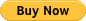Search

# 29 – All About Margin Level in a Margin Account

Margin level is a concept which goes along with Equity and Used Margin. So, it is recommended to have a look back at the previous lessons before getting into this lesson.

#### What is Margin Level?

Margin Level is the term expressed in percentage, which is based on the amount of Equity vs Used Margin.

In simple terms, Margin Level tells you how much of your funds are available for trading. The higher the Margin Level, the more is the amount (Free Margin) available for you to take trades. Similarly, lower the Margin Level, lesser is the Free Margin. Moreover, a significant decrease in the Margin Level could lead to something called Margin Call or Stop Out Level, which shall be discussed in the subsequent lessons.

#### Calculating Margin Level

Margin Level is calculated using the simple formula,

Margin Level = (Equity/ Used Margin) x 100%

Knowing your Margin Level is essential because it determines if you can open new positions on your account or not.

#### Comprehending Margin Level

When there are no trades open, the Margin Level will be equal to zero. And when there are running positions, the Margin Level will be expressed in percentages depending on the values of Equity and Used Margin.

Typically, brokers set the limit of Margin Level to 100%. That is, if your Margin Level falls below 100%, you will not be able to place any more additional trades. And if you wish to open a new position, you must close the existing trade first.

Let us get a grip on the concept by taking an example.

Example: USD/CHF

Let’s say you have deposited \$1,000 in your margin account and you wish to go long 10,000 units on USD/CHF.

To calculate the Margin Level, we must first calculate the parameters such as Required Margin, Used Margin, and Equity.

Required Margin

Assuming the Margin Requirement to be 2%, the Required Margin can be calculated as,

Required Margin = Notional Value x Margin Requirement

= \$10,000 x 2% (0.02)

= \$200

Considering the account is dominated in USD, the Required Margin will be \$200.

Furthermore, since there is only one position open, the Used Margin will be equal to the Required Margin.

Equity

Equity is the sum of the Account Balance and Floating profits/losses. Assuming the position to be at breakeven, the Equity turns out to be,

Equity = Account Balance + Floating P/L

= \$1,000 + \$0

= \$1,000

Hence, the Equity value is \$1,000.

Now, that we have all the required variables, let us go ahead and calculate the Margin Level.

#### Margin Level

Margin Level = (Equity / Used Margin) x 100%

= (\$1,000 / \$200) x 100%

= 500%

Therefore, the Margin Level on this account will be 500%.

And since this percentage is above the 100% mark, most brokers will allow you to take new positions.

Thus, this completes the lesson on the Margin Levels.

See All

## Credit

Caution: